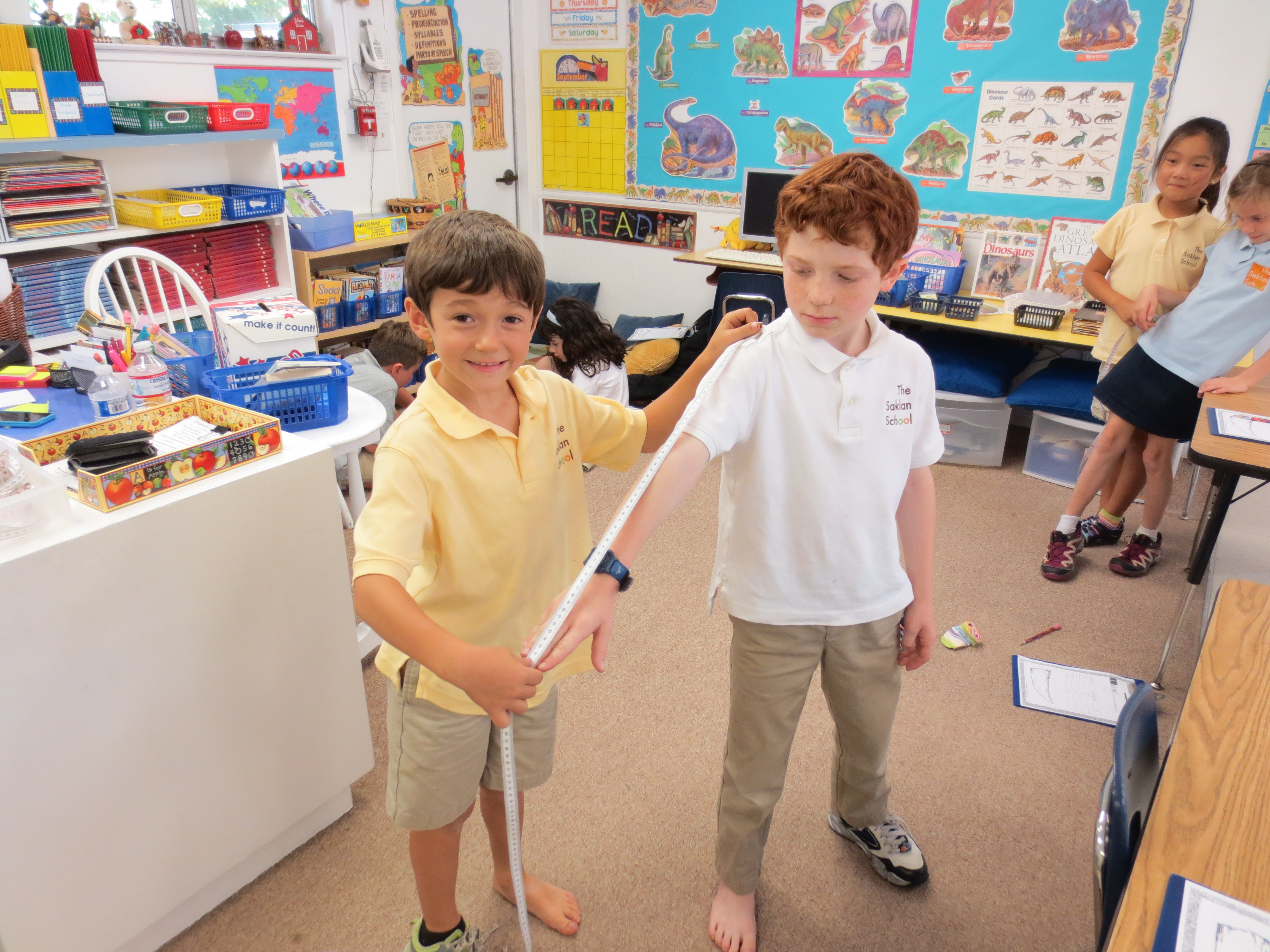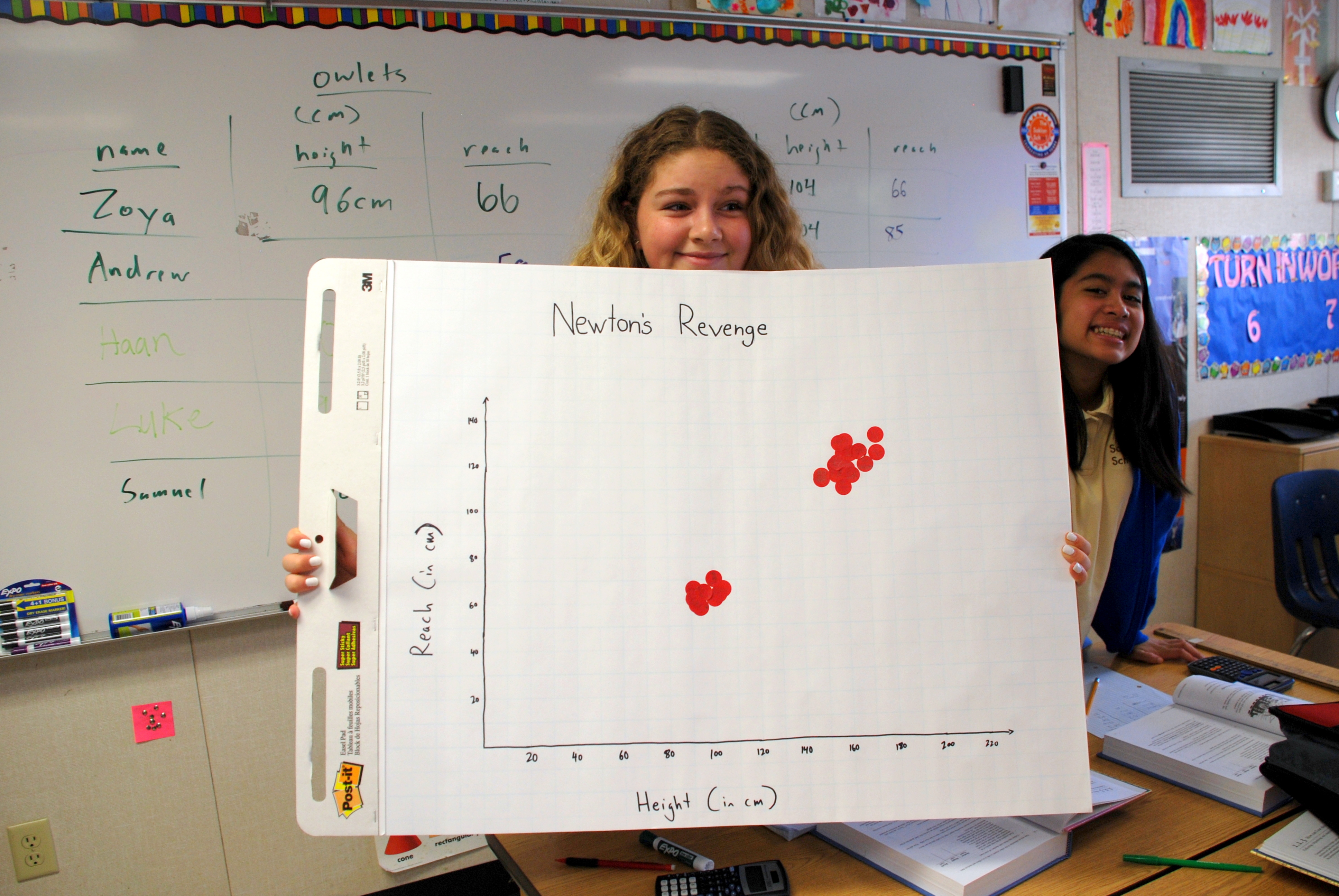# The Saklan School# Math K-8

Math K-5

OverviewUsing the Sadlier-Oxford Progress in Mathematics Program, our math curriculum connects mental math and logical reasoning skills to mathematical concepts and applications. Curriculum standards are set for each grade level to align and surpass California state and national standards. The curriculum developmentally spirals and teachers create meaningful learning opportunities though manipulative, cooperative learning, problem-solving, and real-life applications.

Kindergarten
Mathematical concepts are learned through hands-on exploration. Emphasis is placed on number concept, patterns, counting, addition and subtraction, graphing, classifying, sorting, place value, money, time, number writing, and shapes.

Math is integrated throughout the day from morning calendar activities to various projects that require counting, measuring, estimating and other skills. First grade focuses on place value, graphing, algebra, geometry, addition and subtraction facts through double digits up to 99 and problem solving using various strategies. Skills are practiced using manipulatives, group, and independent practice.

Math is integrated into all subjects. Students learn place value, fact families, computation and regrouping (+/-) up through four digits, graphing, fractions, money, time, geometry, estimation, algebra and measurements. Single digit multiplication and division up through at least 5 is also taught. Special attention is given to critical thinking and problem solving.

The focus is on problem solving approaches emphasizing strategies, applications and problem formulation. Students learn computation & regrouping, double digit multiplication and division up to 12, decimals and fractions, geometry, fractions, statistics & probability, graphing, estimation and algebraic applications. Students use hands-on activities, mathematical projects & manipulatives that make math fun and memorable.

Students work on numeration, computation, and regrouping numbers through the millions. They multiply and divide multiple digits, add and subtract fractions and decimals. Fourth grade math gives students a greater understanding of measurement techniques, geometry, and algebraic thinking. Students learn the standard and metric measurement units, learn to graph and interpret data using a wide variety of tools.

The emphasis is placed on problem solving strategies, where students work independently, as partners, and in groups to develop critical thinking skills. Areas of emphasis include addition, subtraction, multiplication and division of whole numbers as well as fractions and decimals, number theory, geometry, measurement, graphs, data, and analysis. Probability, proportion, percentage, and statistics are also studied.

Middle School Math

OverviewAll Middle School Math classes use the inquiry based College Preparatory Math (CPM) Curriculum. Students explore mathematical ideas in ways that maintain their enjoyment of and curiosity about mathematics, help them develop depth of understanding, and reflect real-world applications. To become good problem solvers, students need many opportunities to create and solve problems in both mathematical and real-world contexts. They also need to develop and build upon a strong number sense. This involves posing questions, defining problems, considering different strategies, and finding appropriate solutions. Working together in teams and groups enhances mathematical learning, helps students communicate effectively, and develops social and mathematical skills.  Below are the course descriptions:

Pre-Algebra, Making Connections
Students spiral through many topics in this first year of Pre-Algebra, but each area is connected in a logical manner to enhance student learning.  Some of what they are exposed to include patterns, proportional relationships, comparisons, data collection and analysis, equivalent fractions, writing expressions with variables, probability, volume, area, prisms, dividing fractions, percent applications, and coordinate graphing.

Pre-Algebra, Making Connections, Year 2
Students will continue to revisit the concepts introduced in the first year of Pre-Algebra, while they fine tune their skills and go further in depth during year 2. New topics include the Pythagorean Theorem, solving inequalities, linear and exponential growth, scientific notation, interest, slopes, solving equations, exponent rules, cones and pyramids, scale factor, circles, solving complex word problems, and transformations.

Algebra
This course is a thorough introduction to algebraic techniques and their applications. Basic algebraic skills will be emphasized, with some use of the graphing calculator. Topics include linear, exponential, and quadratic functions, along with polynomials, factoring and radicals.

Geometry
This course is designed for advanced 8th graders and is a high school level geometry class. Rigorous proofs, properties and relationships of plane figures, and trigonometric ratios taught in a challenging manner? The course is structured around problems and investigations that build spatial visualization skills, conceptual understanding of geometry topics, and an awareness of connections between different ideas. Students are encouraged to investigate, conjecture, and then prove to develop their reasoning skills.